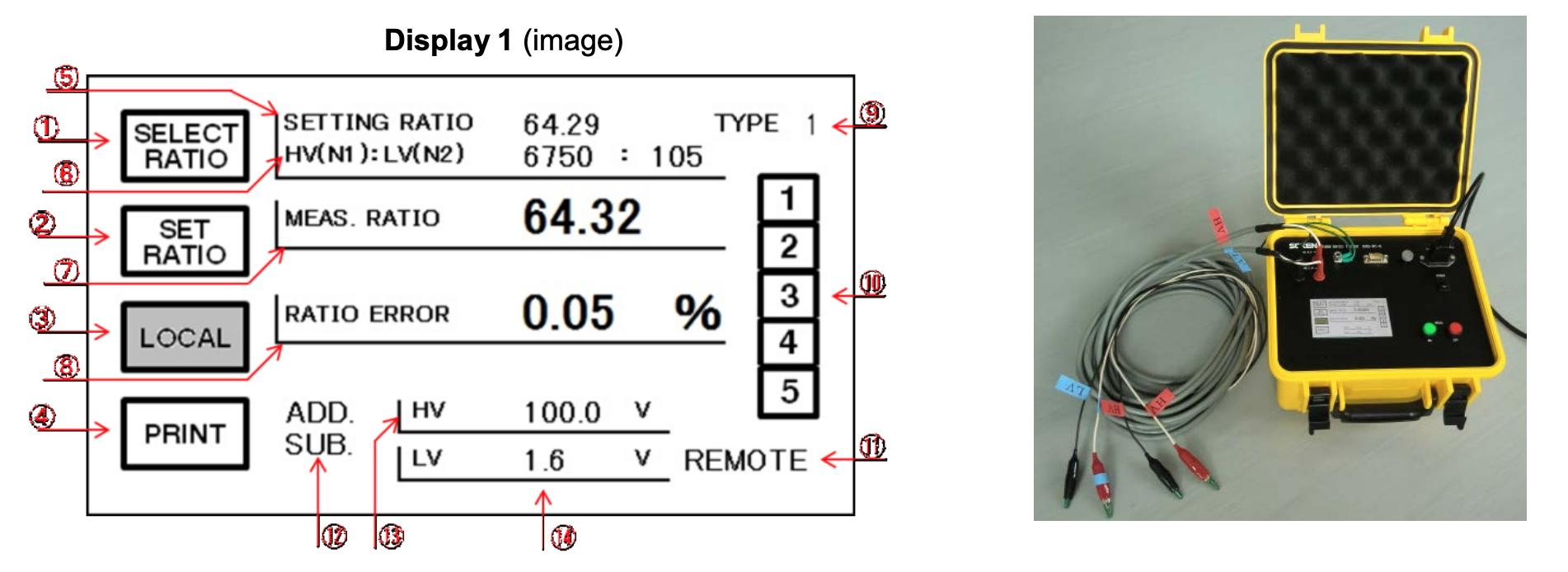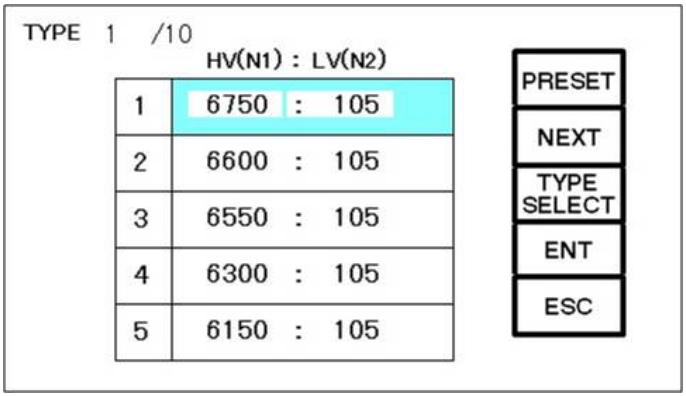Cart : (empty)

# Soken DAC-RT-6 - Turn Ratio Tester

## Category :Instrument Transformer Testing Set

### by quote onlyAdd to Cart

Documents:

Overview
Features
Information
Calibration

DAC-RT-6 is designed to measure winding turns ratio between the primary and secondary winding of a
power transformer and an instrument transformer, preciously.

Built-in amplifier power source realizes stable measurement without interference by the harmonic current in
power-line.

Further, a preliminary measurement (for 10 sec.) is given before the main measurement to secure safety.
In case of detecting the wrong connection between primary and secondary windings, or any miss setup of
turn ratio etc, the tester stops measurement with an alarm sound.

Moreover, to pursue usability for users, max 10 sample (Turns ratio: 5 points for every sample) can be
registered in internal memory.

DAC-RT-6 is an ideal tester for safe, accurate, and efficient measurement of winding turns ratio.

## Specimens

Power Transformers, Instrument Transformers

Principal

Winding turns ratio error means how much real turns ratio (TRn) is different from the nominal turns ratio (TR), and is calculated by using the following formula.

ε= (TR-TRn) / TRn × 100 %

Turns Ratio: TR
Nominal Turns Ratio: zTRn
Turns-Ratio Error: ε

DAC-RT-6 applies test voltage (100V) to the HV side of a transformer under test and detect generated voltage at the LV side. Turns ratio error will be measured by comparing the nominal turns ratio value. Polarity (ADD or SUB) is discerned from phase differences between the HV side and the LV side. The true turns ratio value will be displayed by calculating the measured ratio error and the nominal turns ratio value.

## Display1. SELECT RATIO: Move to the ratio selection screen
2. SET RATIO: Move to ratio setting screen
3. LOCAL: Release REMOTE condition
4. PRINT: Print out from an option printer
5. SETTING RATIO: Turns ratio value calculated from HV(N1) and LV(N2).
6. HV(N1): LV(N2): Set-up values of HV(N1) and LV(N2)
7. MEAS. RATIO: Measured turns ratio
8. RATIO ERROR: Measured turns ratio error
9. TYPE: Move to list of the transformer (display 2)
10. Tap number: Select registered ratio
11. REMOTE: Shown when the tester is in Remote mode
12. POLARITY: Discerned polarity (ADD or SUB)
13. HV: Voltage applied to N1 H.V
14. LV: Voltage generate to N2 L.V

### Display 2(image)Max 10 transformer types can be registered and numbered from 1 to 10, and Max 5 ratios can be registered for every type. Each of 5 ratios is displayed on a screen and can be selected by touching the number from 1 to 5. (Ref. display 2)

Soken DAC-RT-6 - Turn Ratio Tester
• Preliminary measurement function to secure safety. (Stop measurement with alarm sound at any abnormal condition)
• The built-in amplifier power source prevents interference from harmonic current in Power-line.
• Max 10 types of turns ratio can be registered, and 5 different ratio settings are available for every type. (totally 50 settings)
• Durable, compact, and lightweight.
• Simple operation with an LCD screen.

## General

Measuring VoltageAC100V (20VA)
Turns Ratio Range0.8:1 to 1:1500
Ratio Error Rate Range
 ± 2% range 0 - ±2.00% ± 20% range 0 - ±20.00%
Accuracy
Turns Ratio < 200
 Range: 2% Accuracy: ± 0.05% ± 2 digits Range: 20% Accuracy: ± 0.1% ± 2 digits
Turns Ratio 201 - 1000
 Range: 2% ~ 20% Accuracy: ± 0.1% ± 2 digits
Turns Ratio 1001 - 1500
 Range: 2% ~ 20% Accuracy: ± 0.3% ± 2 digits
Setting Range
 HV(N1) 0.001 – 99999 LV(N2) 0.1 – 9999
Input PowerAC100V-240V, 50/60Hz
InterfaceRS232C
Size (mm)W270 x H180 x D260
WeightApprox. 5kg
AccessoryMeasuring Cable (H.V/L.V) (5M) AC Cord (1.5M), Grounding Cable (3M)

## Do you have a Turn Ratio Tester that needs to be calibrated?

We offer standard traceable calibration and ISO 17025 accredited calibration services.